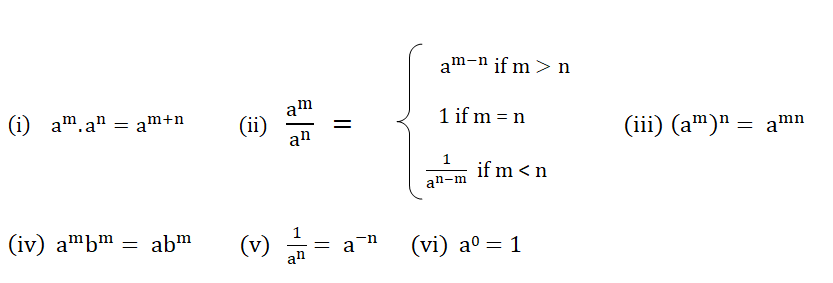Welcome To Basics In Maths

# TS 9th Class Maths concept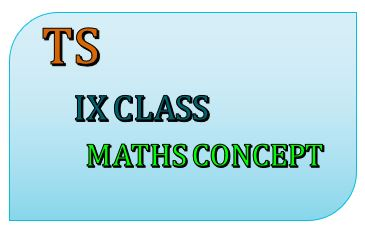Studying maths in IX class successfully means that children take responsibility for their own learning and learn to apply the concepts to solve problems.

# This note is designed by the ‘Basics in Maths’ team. These notes to do help students fall in love with mathematics and overcome fear.

## 1.REAL NUMBERS

### Rational numbers:-

ex:-  3/2, 3/5, 2, 1 and so on

• Natural numbers, Whole numbers, and Integers are rational numbers.
• The rational numbers do not have a unique representation.

Representation of rational number: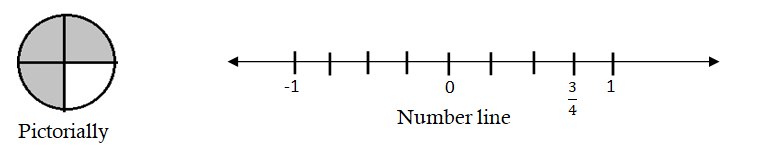To find a rational number between given numbers:

Mean method:- A rational number between two numbers a and b is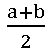Ex:- insert two rational number between 1 and 2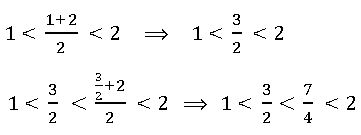To find a rational number in a single step:-

Ex:- insert two rational number between 1 and 2

To find two rational numbers, we 1 and 2 as rational numbers with same denominator 3     (∵ 1 + 2 = 3)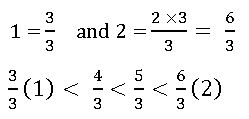The decimal form of rational numbers: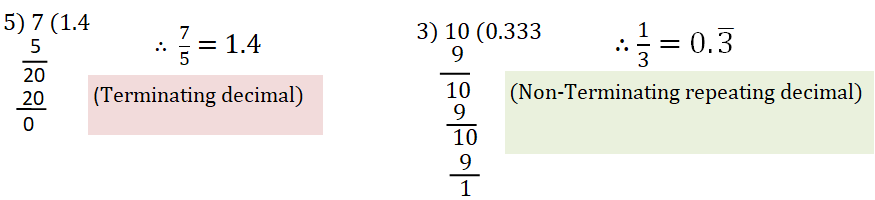• Note:- Every rational number can be expressed as a terminating decimal or non-terminating repeating decimal.
• Converting decimal form into a fraction:
1. Terminating decimals:-  (i) 1.2 = 12/10 = 6/5

(ii) 1.35 =135/100 = 135/100 = 27/20

1. Non-Terminating repeating decimals:-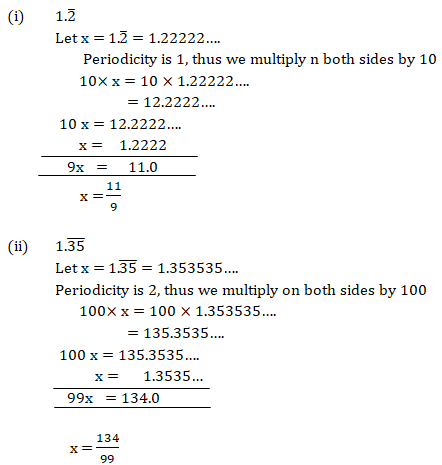### Irrational numbers:

• The numbers which are not written in the form of, where p, q are integers and q ≠ 0 are called rational numbers. Rational numbers are denoted by QI or S.
• Every irrational number can be expressed as a non-terminating decimal or non-repeating decimal.

Calculation of square roots: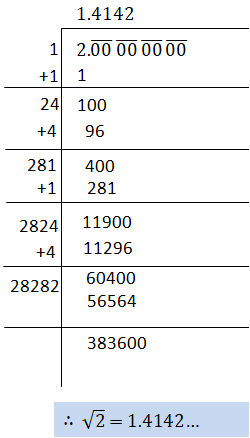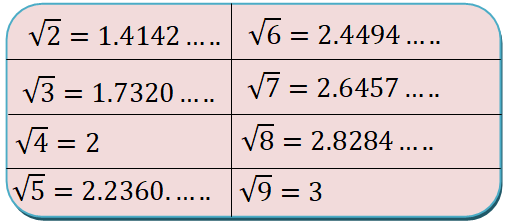#### Representing irrational numbers on a number line:

• At ‘O’ draw a unit square OABC on a number line with each side 1 unit in length.
• By Pythagoras theorem                          OB2 = OA2 + AB2

=  12 + 12

OB2 = 2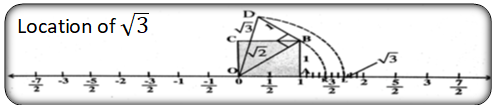• Note:-  If a and b are two positive rational numbers such that ab is not a perfect square, this an irrational number lies between a and b.

### Real numbers

#### Representing real numbers on the number line through successive magnifications:-

locating 2. 775 on a number line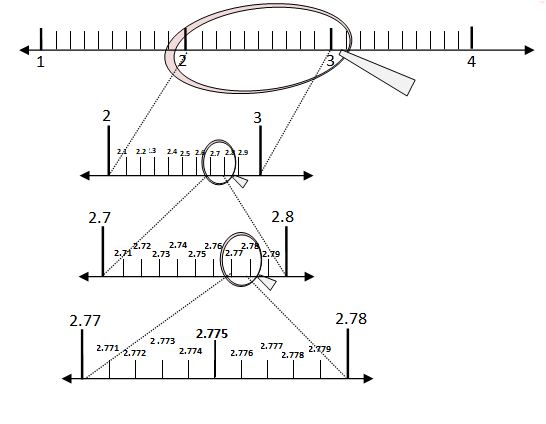### Operation on real numbers

• The sum, difference, product and quotient of irrational numbers need not be an irrational number.
• Irrational numbers are not closed under addition, subtraction, multiplication, and division.
• For any two real numbers a and b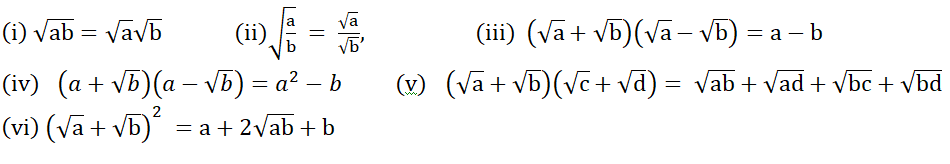#### Rationalizing the denominator:

• Rationalizing factor(R.F):-If the product of two irrational numbers is rational, then each of the two is the rationalizing factor to others.
• The rationalizing factor of a given irrational number is not unique. It is convenient to use the simplest of all R.F.s of given irrational number.
• Note:-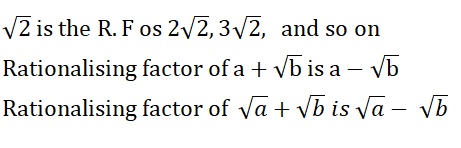### Law of exponents for real numbers: